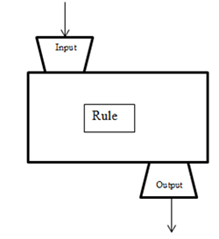# Access Point #: MAFS.912.F-IF.1.AP.2a

Match the correct function notation to a function or a model of a function (e.g., x f(x) y).
Clarifications:

#### Essential Understandings

Concrete:

• Use concrete cause and effect examples (e.g., add blue (x) to yellow [f(x)] to get green (y).
• Use distance (y)/time (x) scenarios where movement f(x) is the function of time (e.g., how long does it take to cross the room).
• Use a function box, e.g.,Representation:
• Recognize in an ordered pair that the first number represents the domain (x-value) and the second number represents the range (y-value).
• Understand function notation (e.g., in y = f(x) is another way to write y is f(x) – read f of x).
• Understand x as the input and y as the output (cause and effect).
• Understand that the graph of f is the graph of the equation y = f(x).
General Information
Number: MAFS.912.F-IF.1.AP.2a
Category: Access Points
Cluster: Understand the concept of a function and use function notation. (Algebra 1 - Major Cluster) (Algebra 2 - Supporting Cluster)

Clusters should not be sorted from Major to Supporting and then taught in that order. To do so would strip the coherence of the mathematical ideas and miss the opportunity to enhance the major work of the grade with the supporting clusters.

## Related Standards

This access point is an alternate version of the following benchmark(s).

## Related Courses

This access point is part of these courses.
1200310: Algebra 1
1200320: Algebra 1 Honors
1200370: Algebra 1-A
1200400: Foundational Skills in Mathematics 9-12
1200300: Basic Mathematics Skills (Formerly Pre-Algebra)
7912070: Access Liberal Arts Mathematics
7912080: Access Algebra 1A
1200315: Algebra 1 for Credit Recovery
1200375: Algebra 1-A for Credit Recovery
7912100: Fundamental Algebraic Skills
1207300: Liberal Arts Mathematics 1
7912075: Access Algebra 1

## Related Resources

Vetted resources educators can use to teach the concepts and skills in this access point.

## Element Cards

High School Math Element Cards:

Element Cards are available to assist in planning for instruction. They are designed to promote understanding of how students move toward the academic standards. Element Cards contain one or more access points, essential understandings, suggested instructional strategies and suggested supports.

Type: Element Cards

## Student Resources

Vetted resources students can use to learn the concepts and skills in this access point.

## Parent Resources

Vetted resources caregivers can use to help students learn the concepts and skills in this access point.